# RS Aggarwal Solutions for Class 7 Maths Chapter 7 Linear Equations in One Variables

RS Aggarwal Solutions for Class 7 Maths Chapter 7 – Linear Equations in One Variable, Pdf is available here for download. Our expert tutors formulate these exercises to assist you with your exam preparation to attain good marks in Maths. Students who wish to score good marks in Maths practise RS Aggarwal Class 7 Solutions. This book is one of the top materials when it comes to providing a question bank to practice.

The main aim is to help students understand and crack these problems. We at BYJU’S have prepared the RS Aggarwal Solutions for Class 7 Maths Chapter 7 wherein problems are solved step by step with detailed explanations. Download pdf of Class 7 Chapter 7 in their respective links.

## Download PDF of RS Aggarwal Solutions for Class 7 Maths Chapter 7 – Linear Equations in One Variables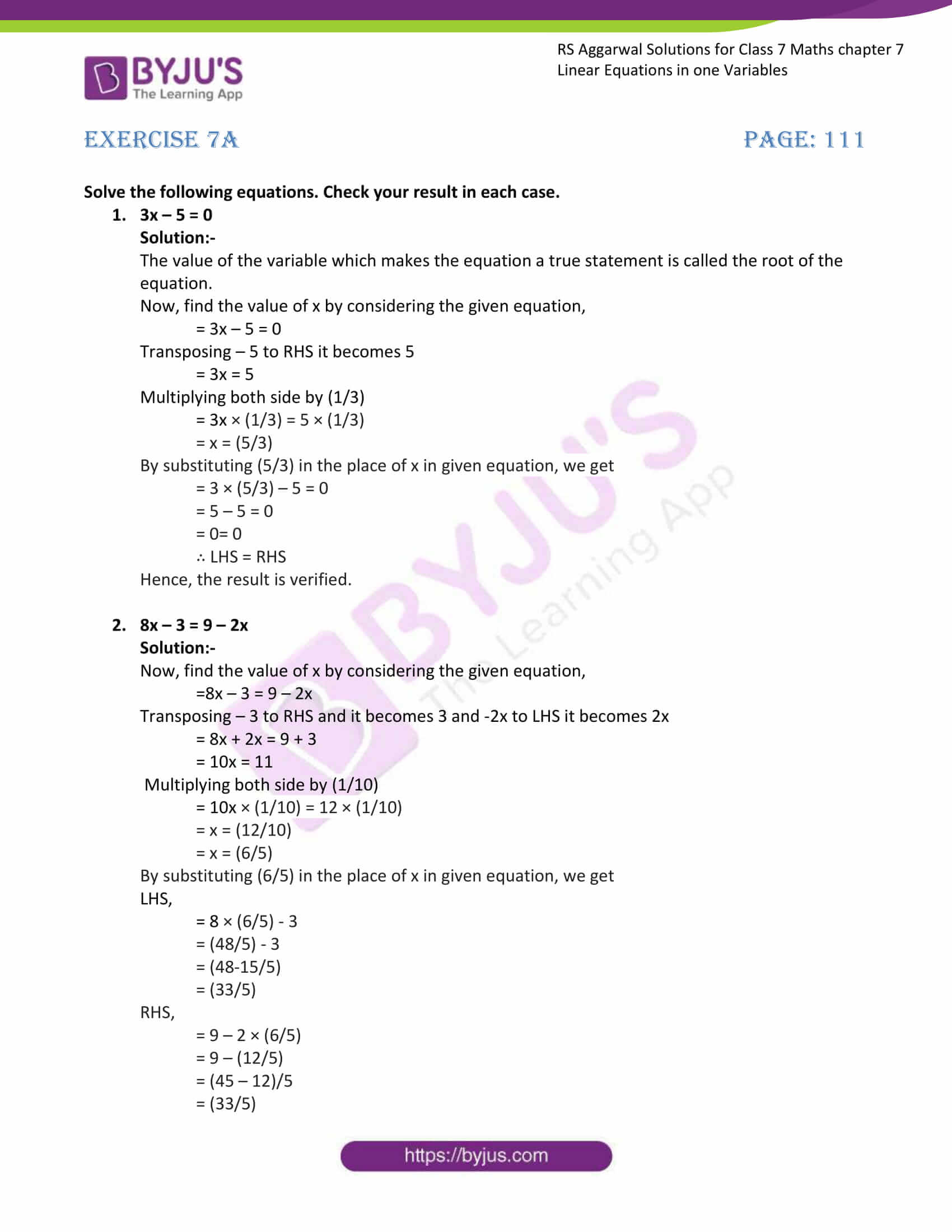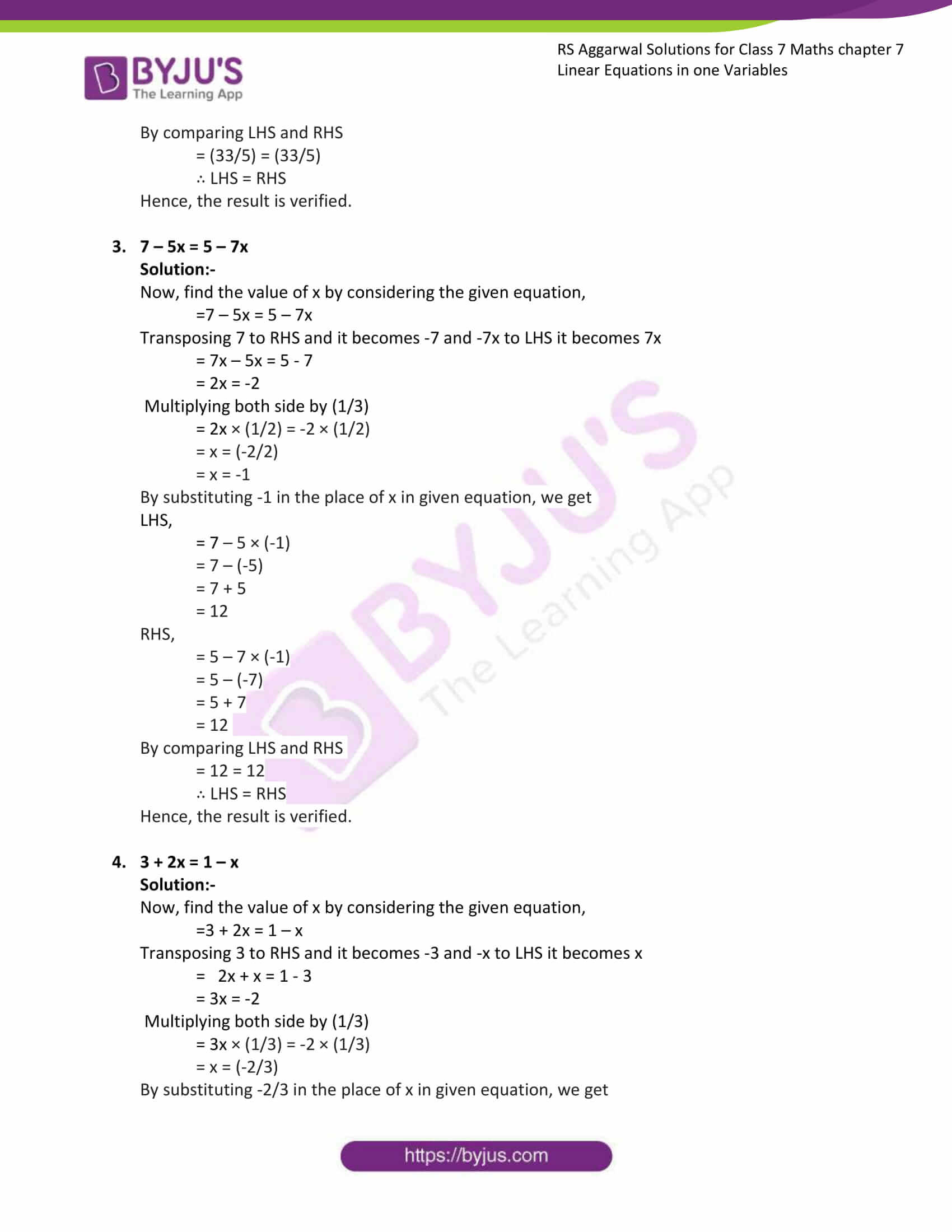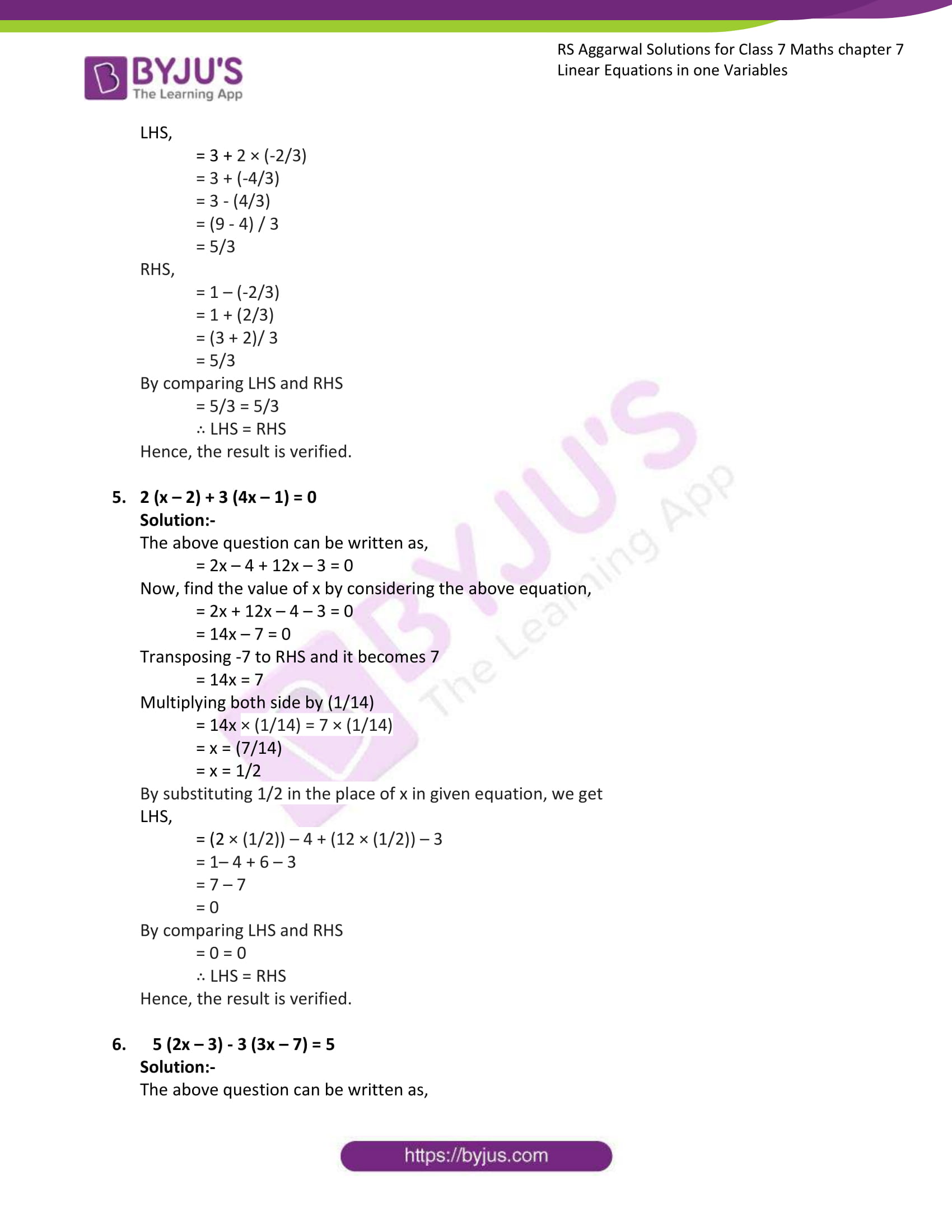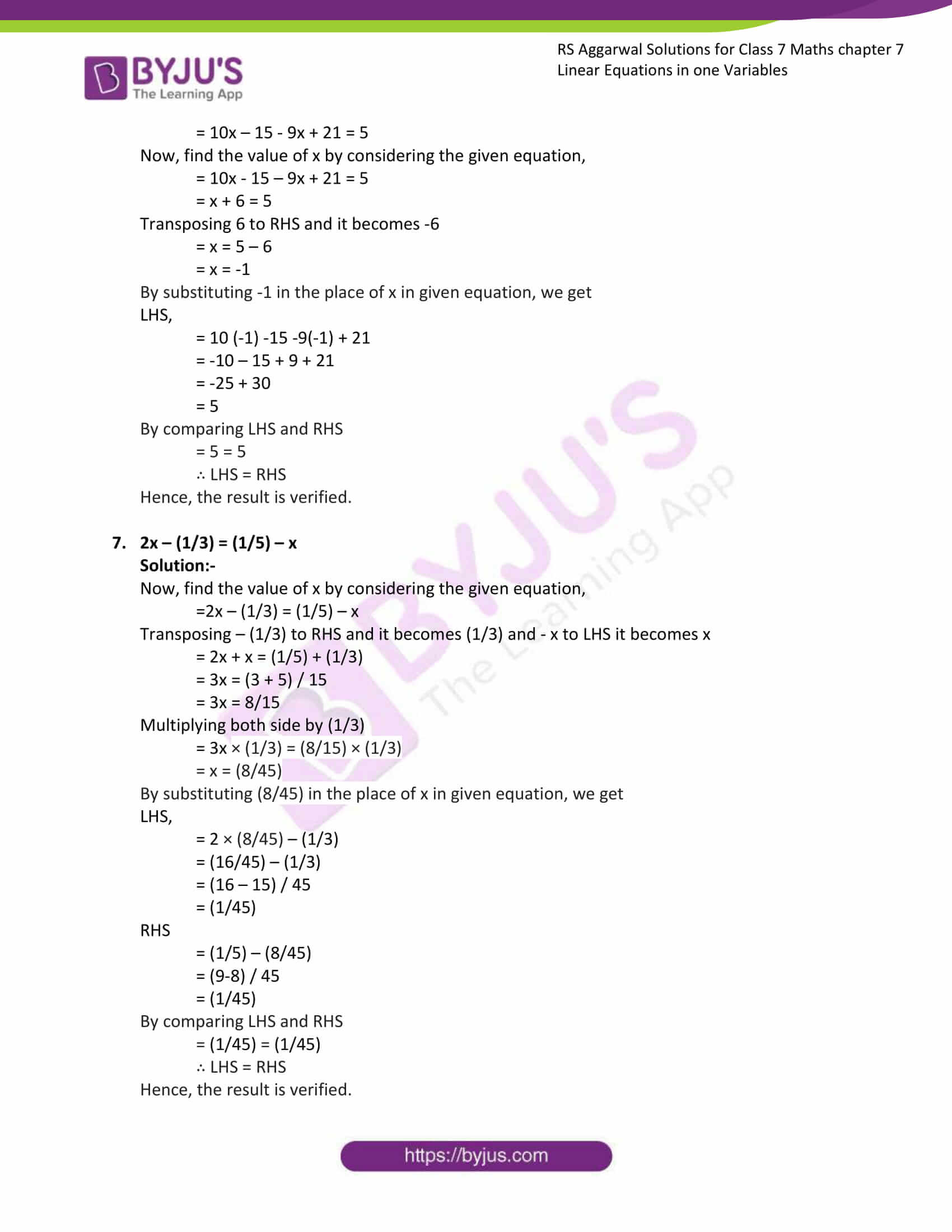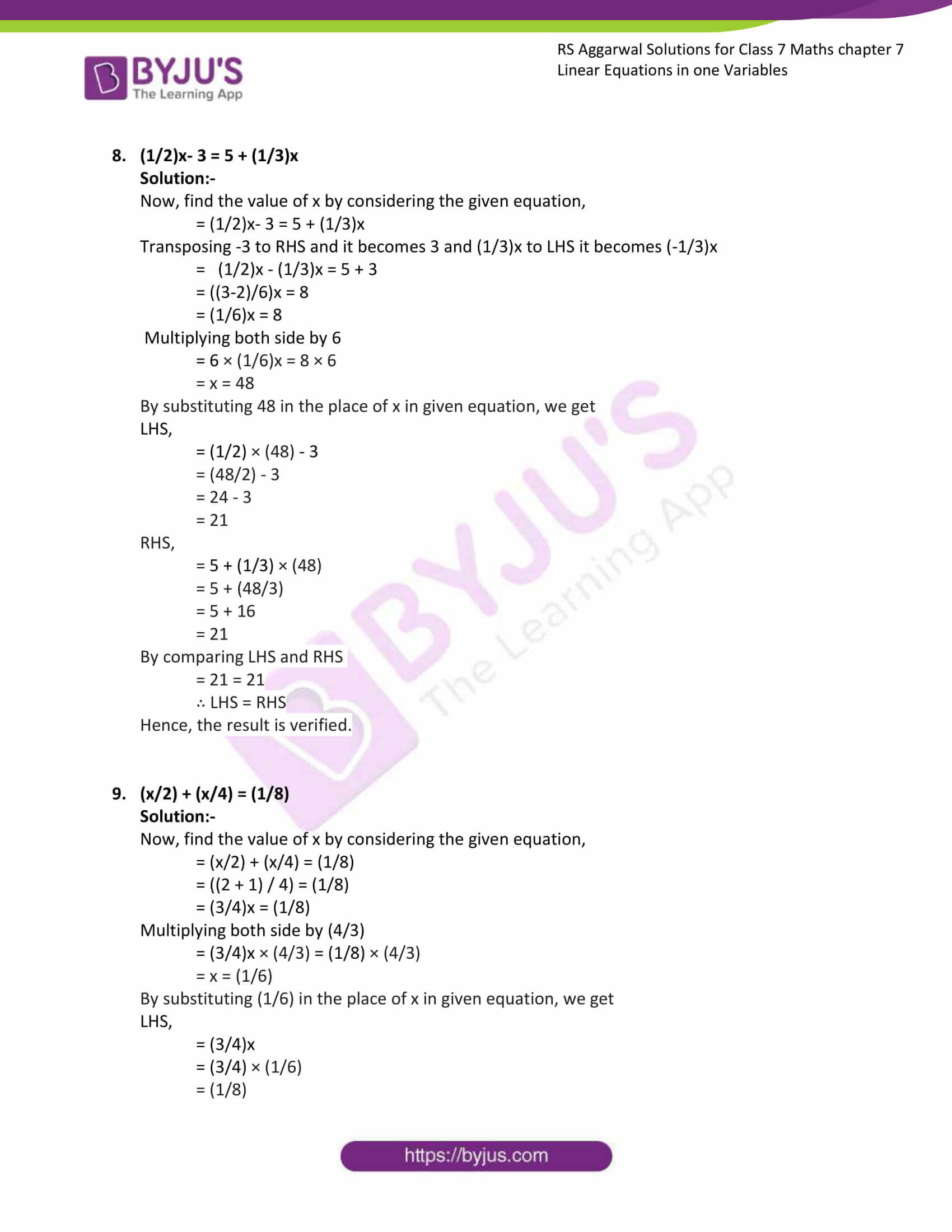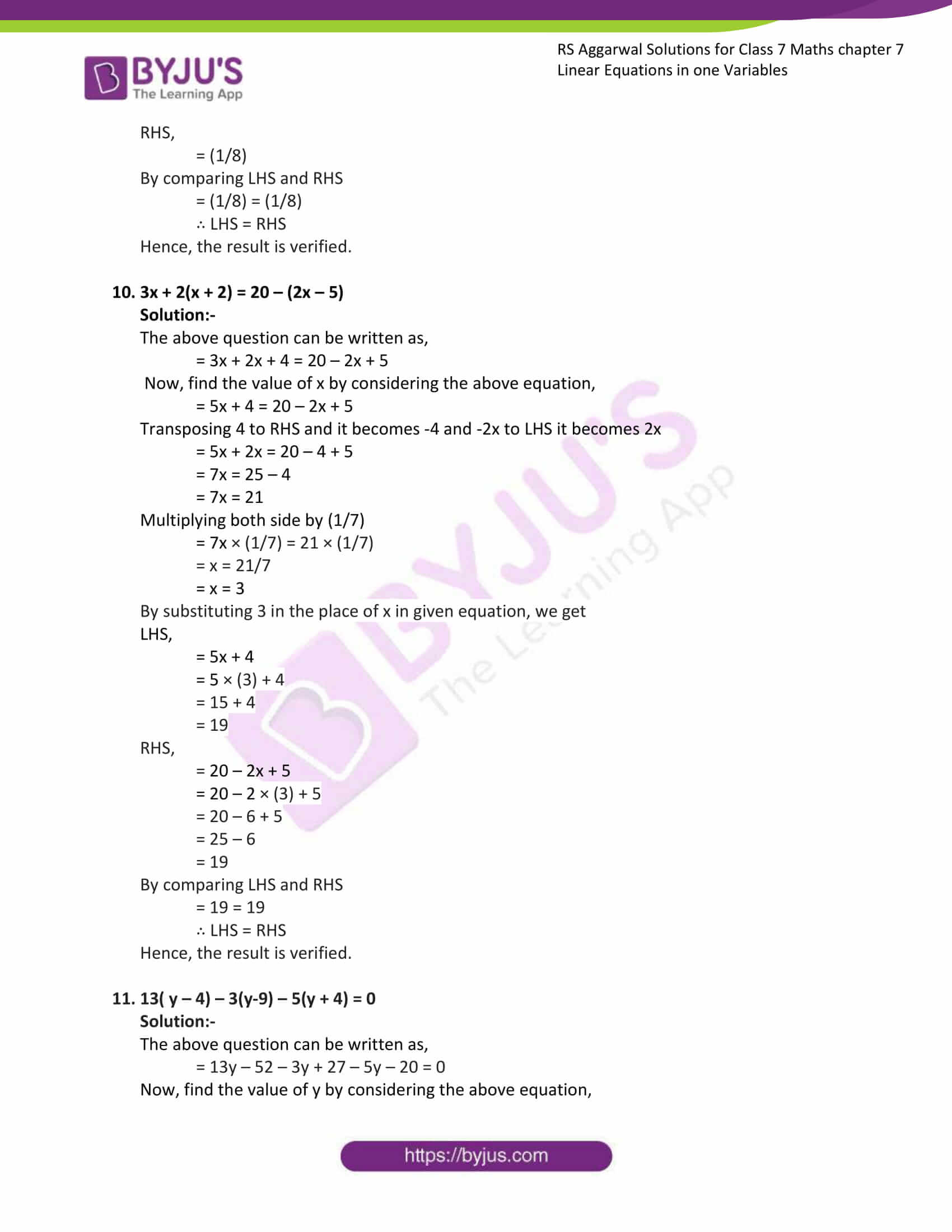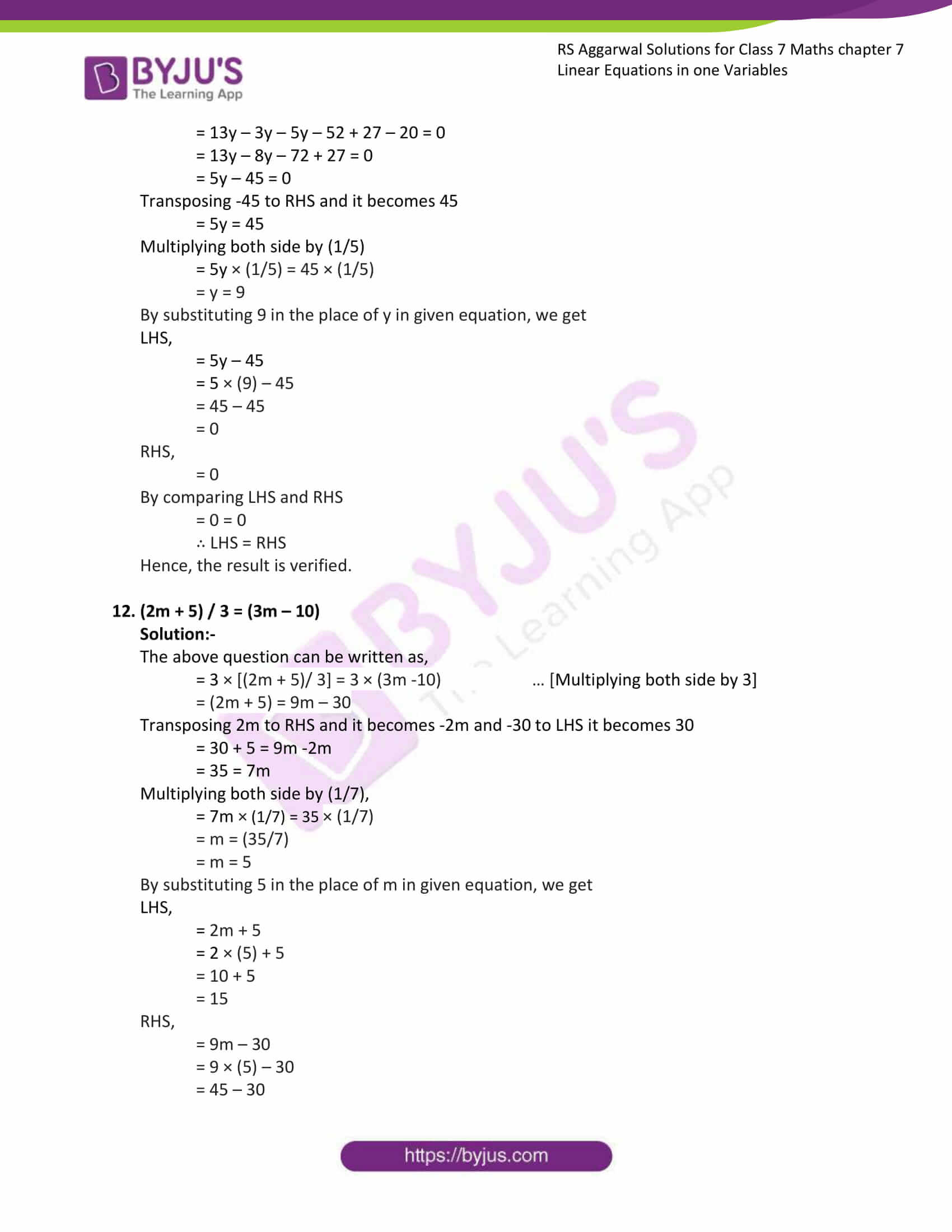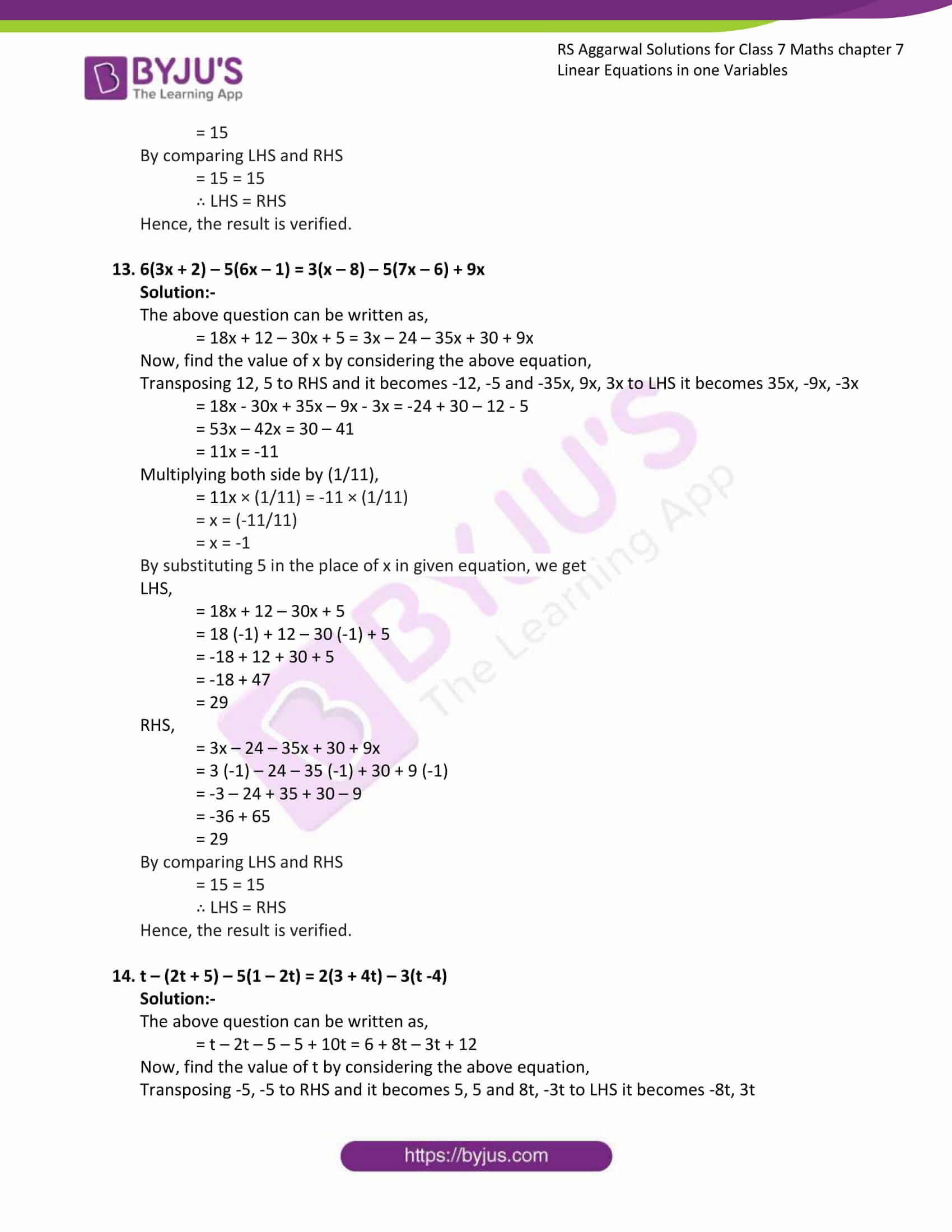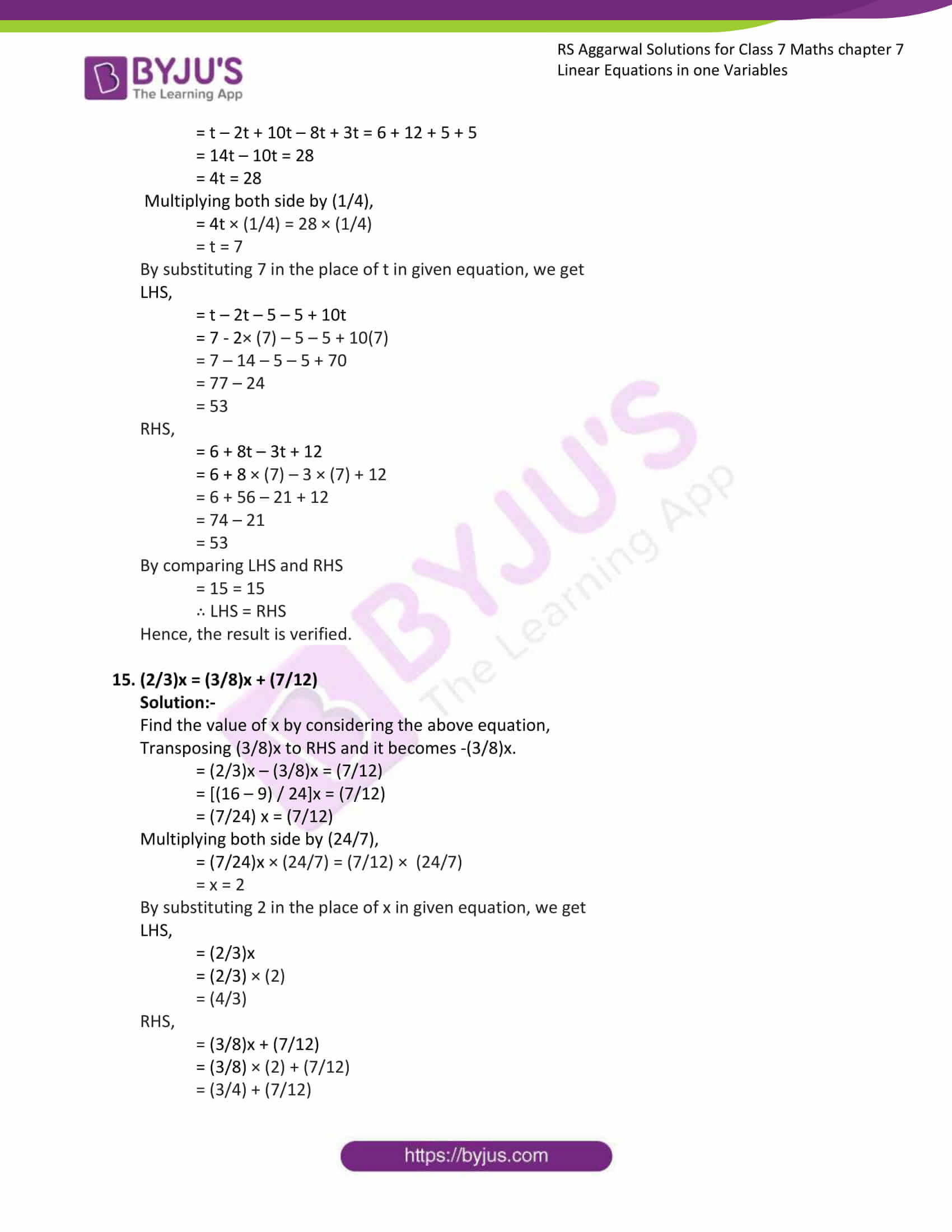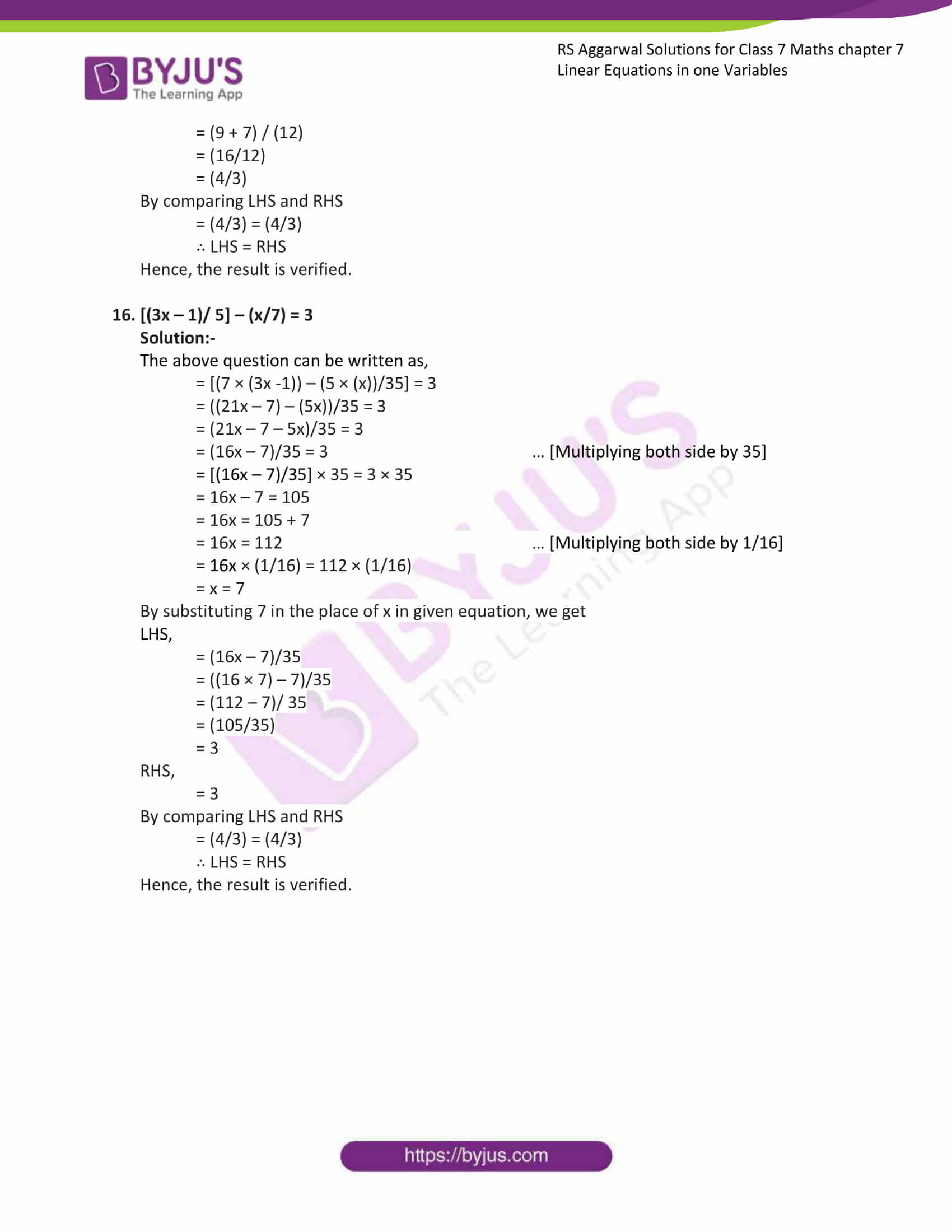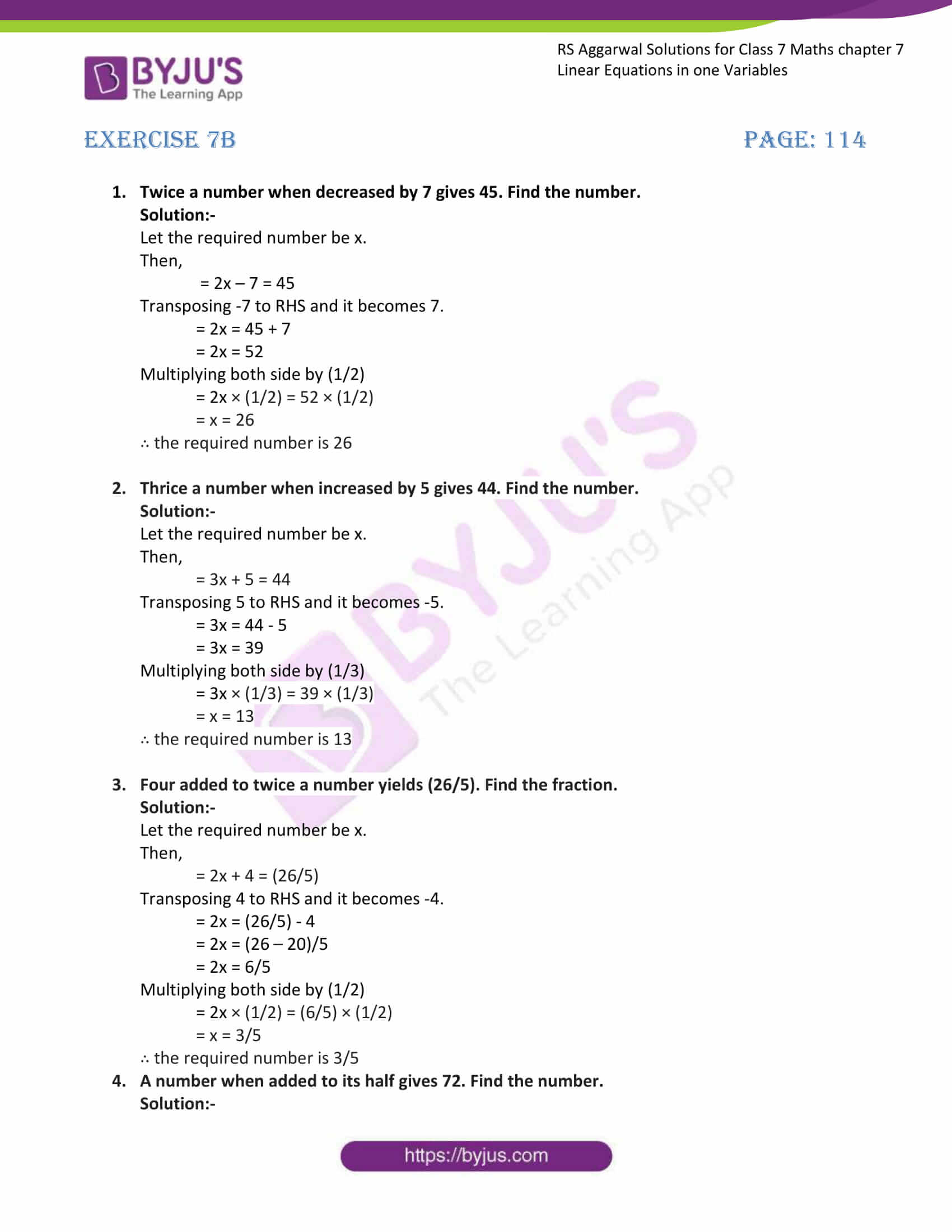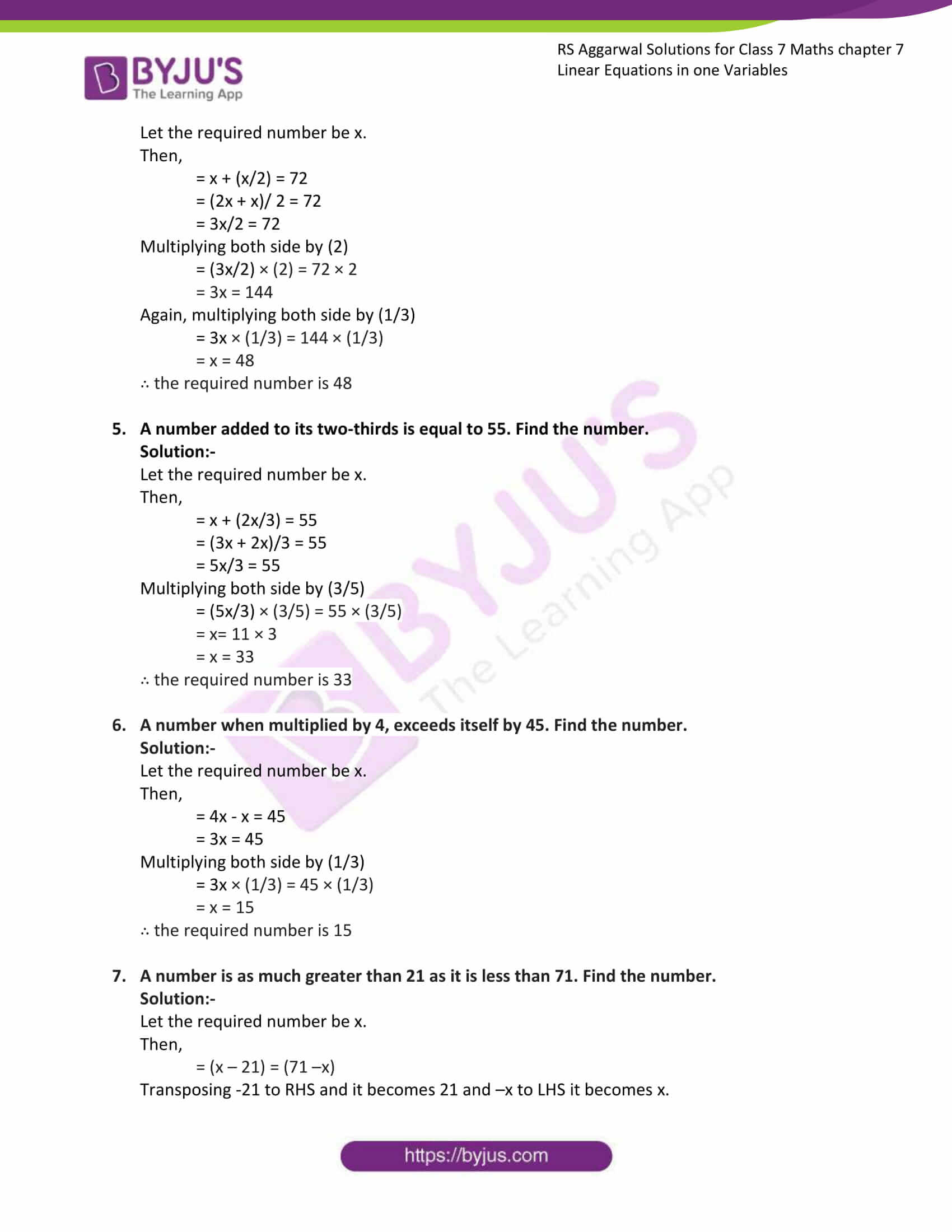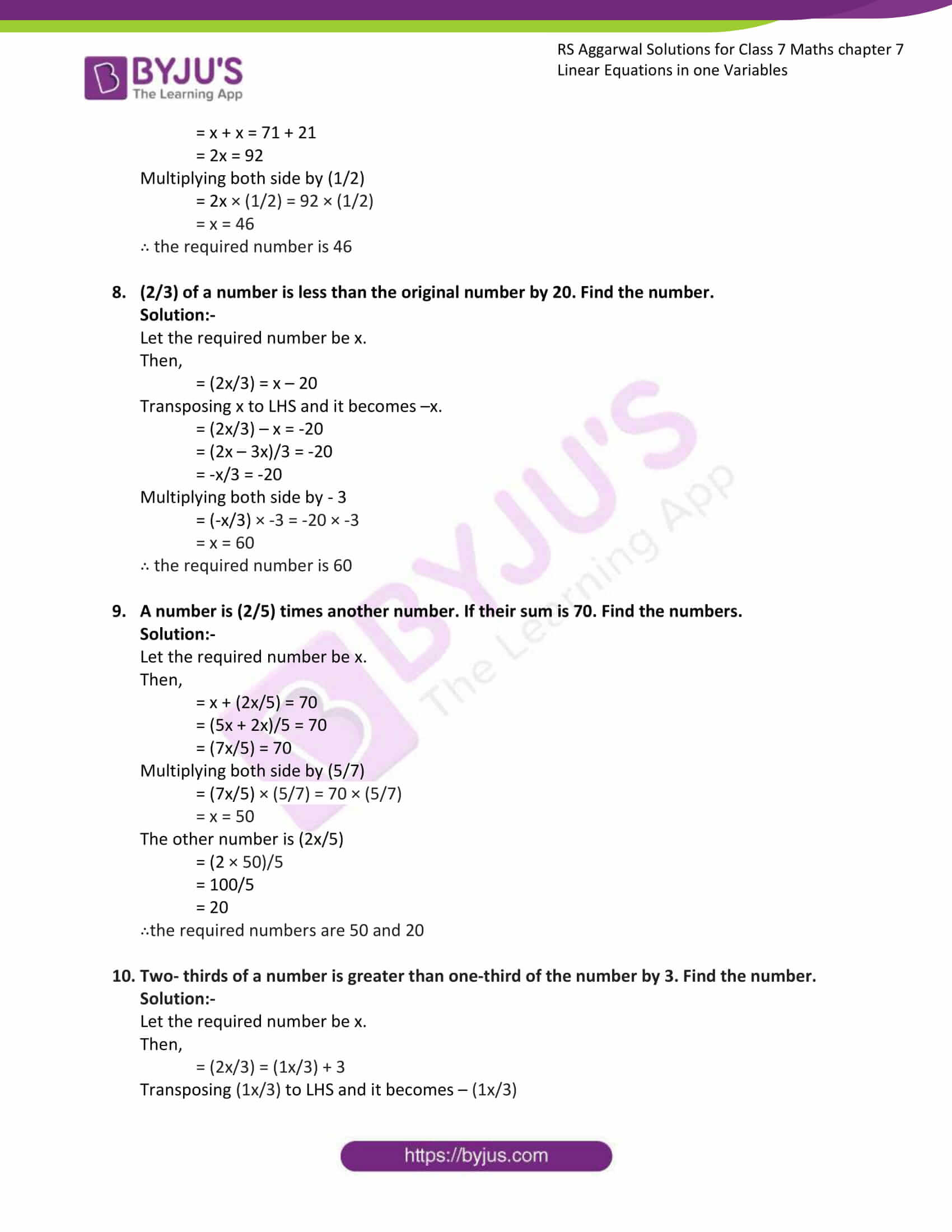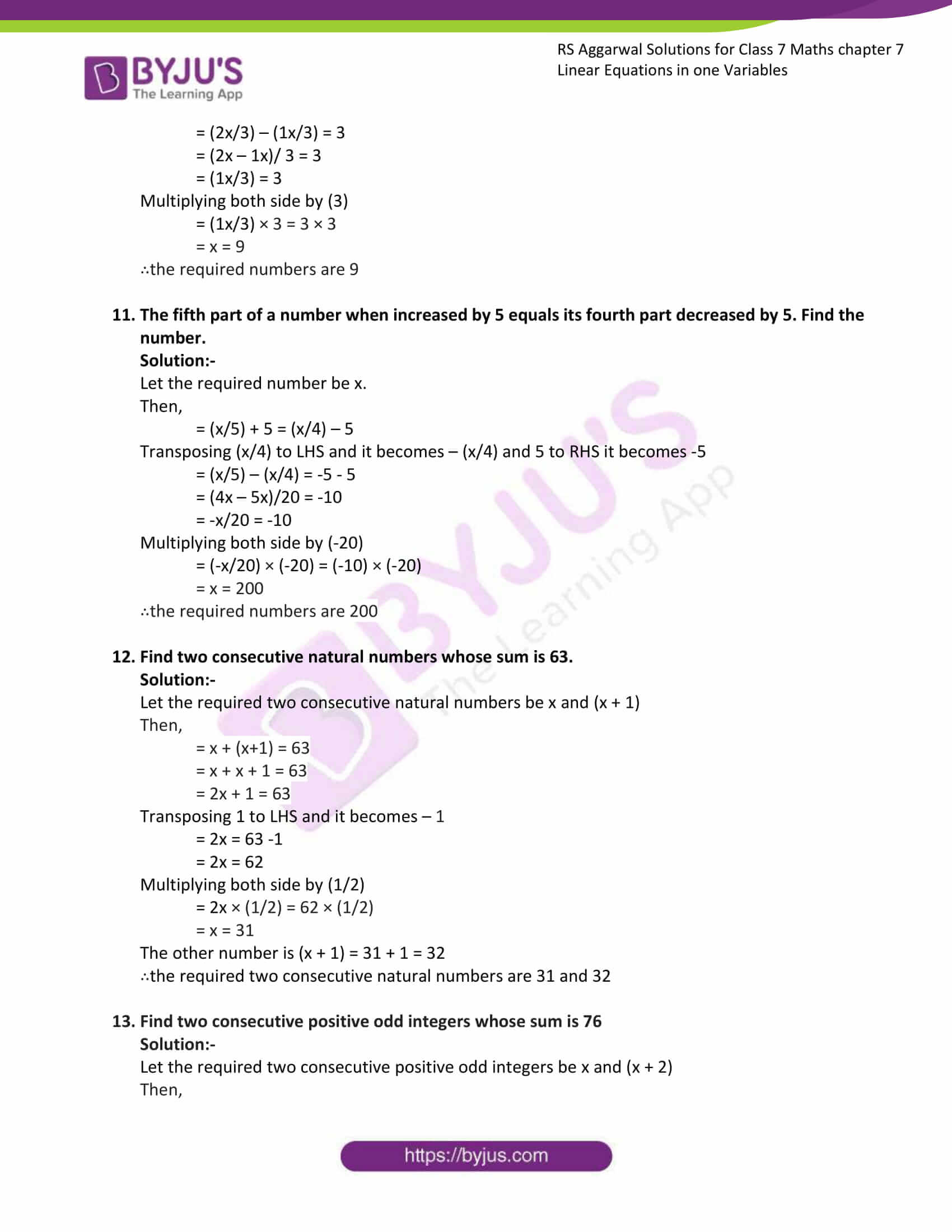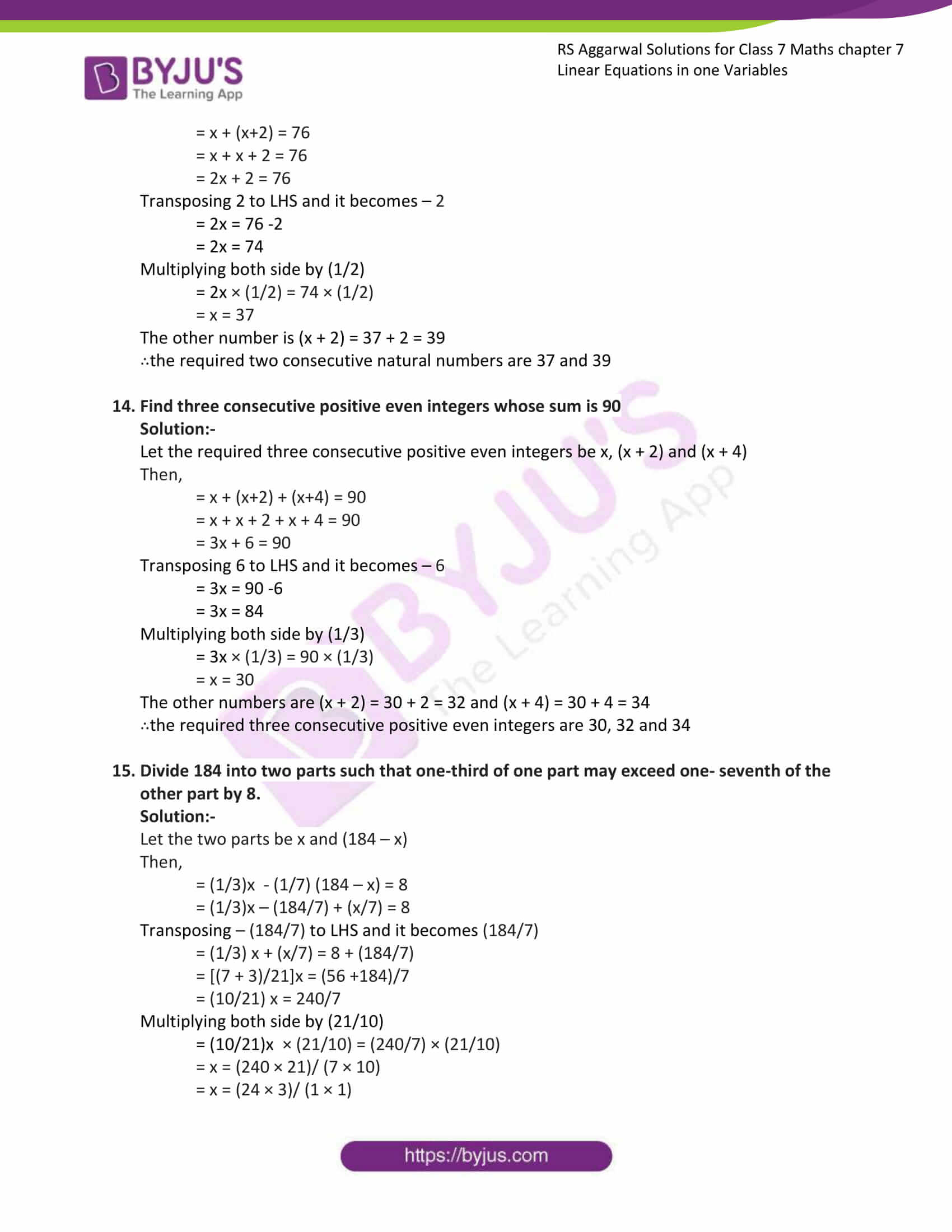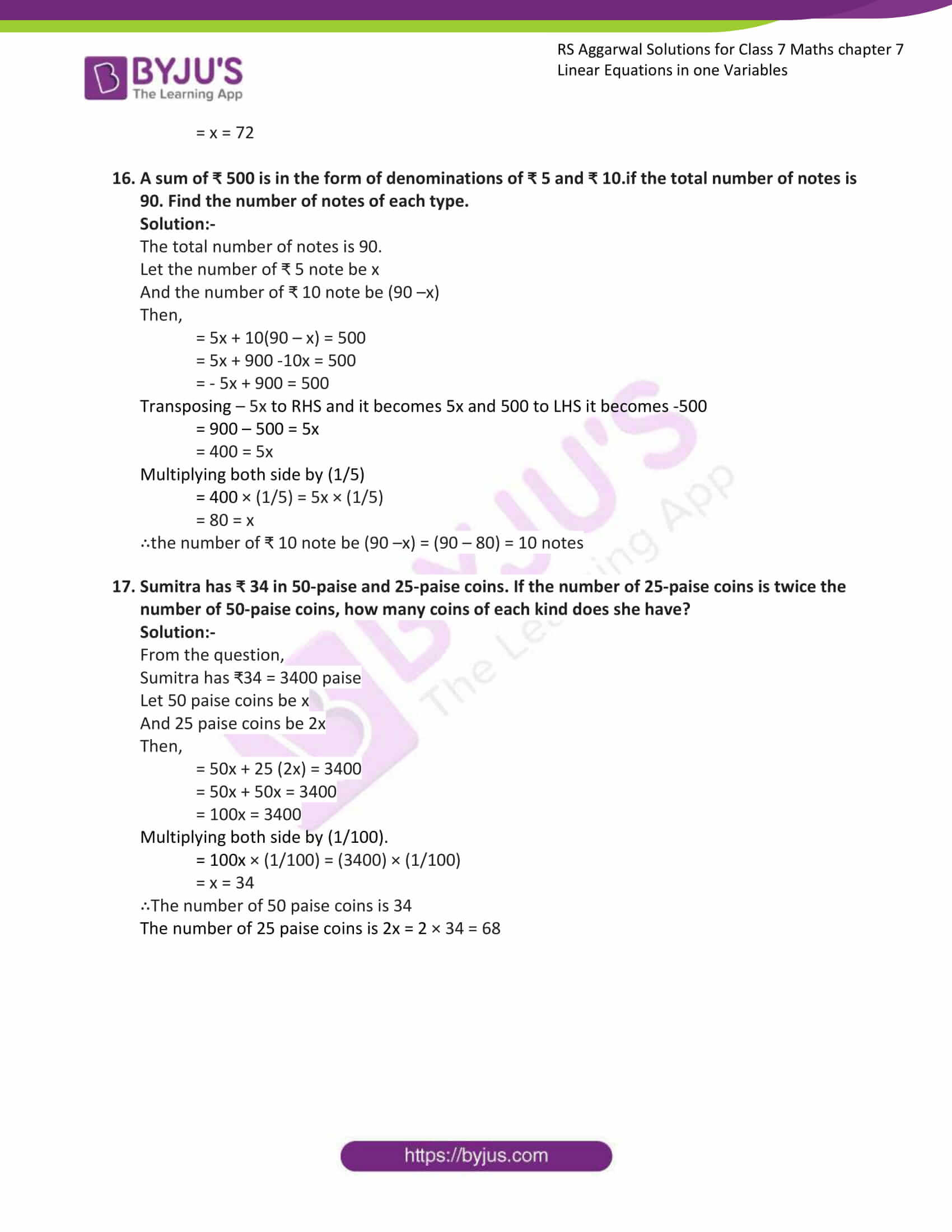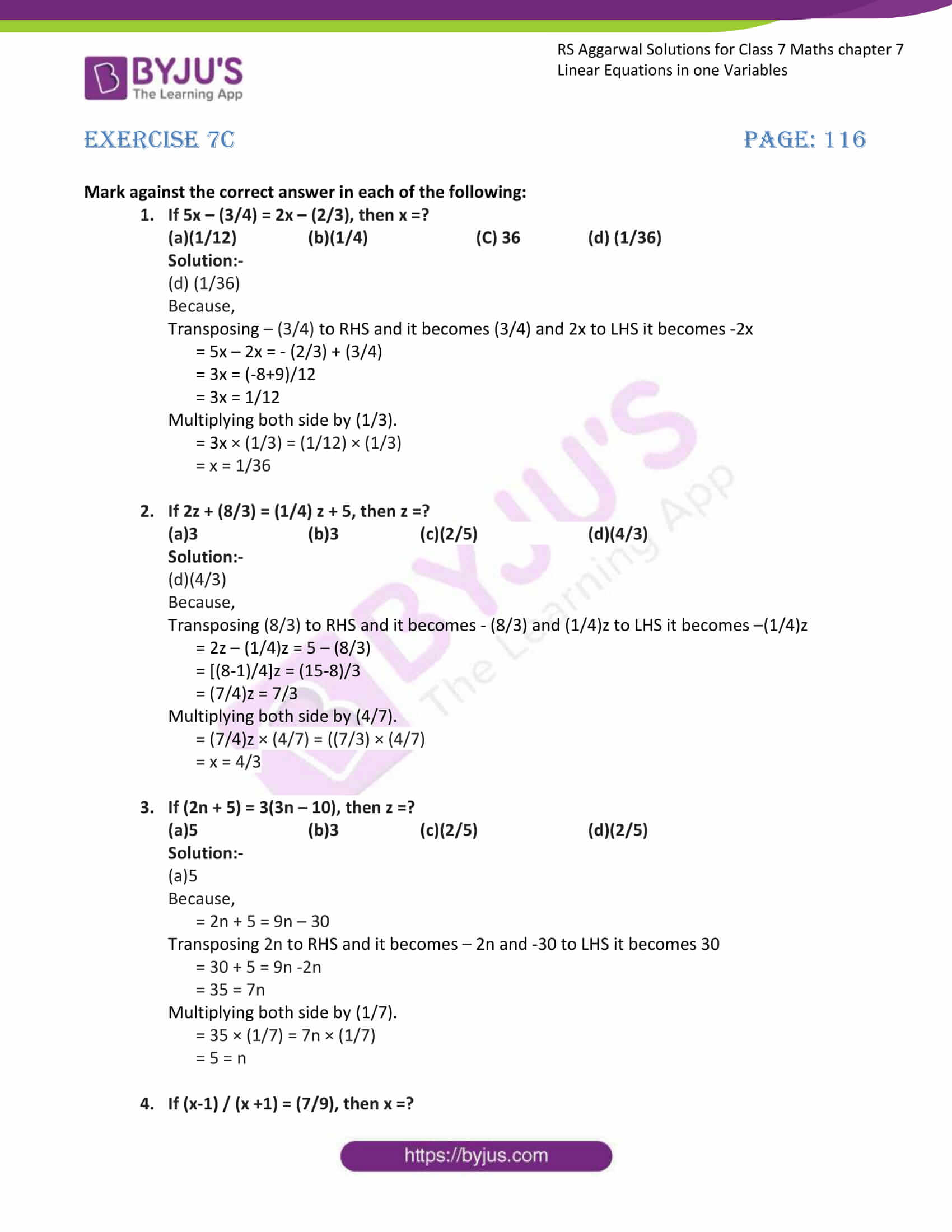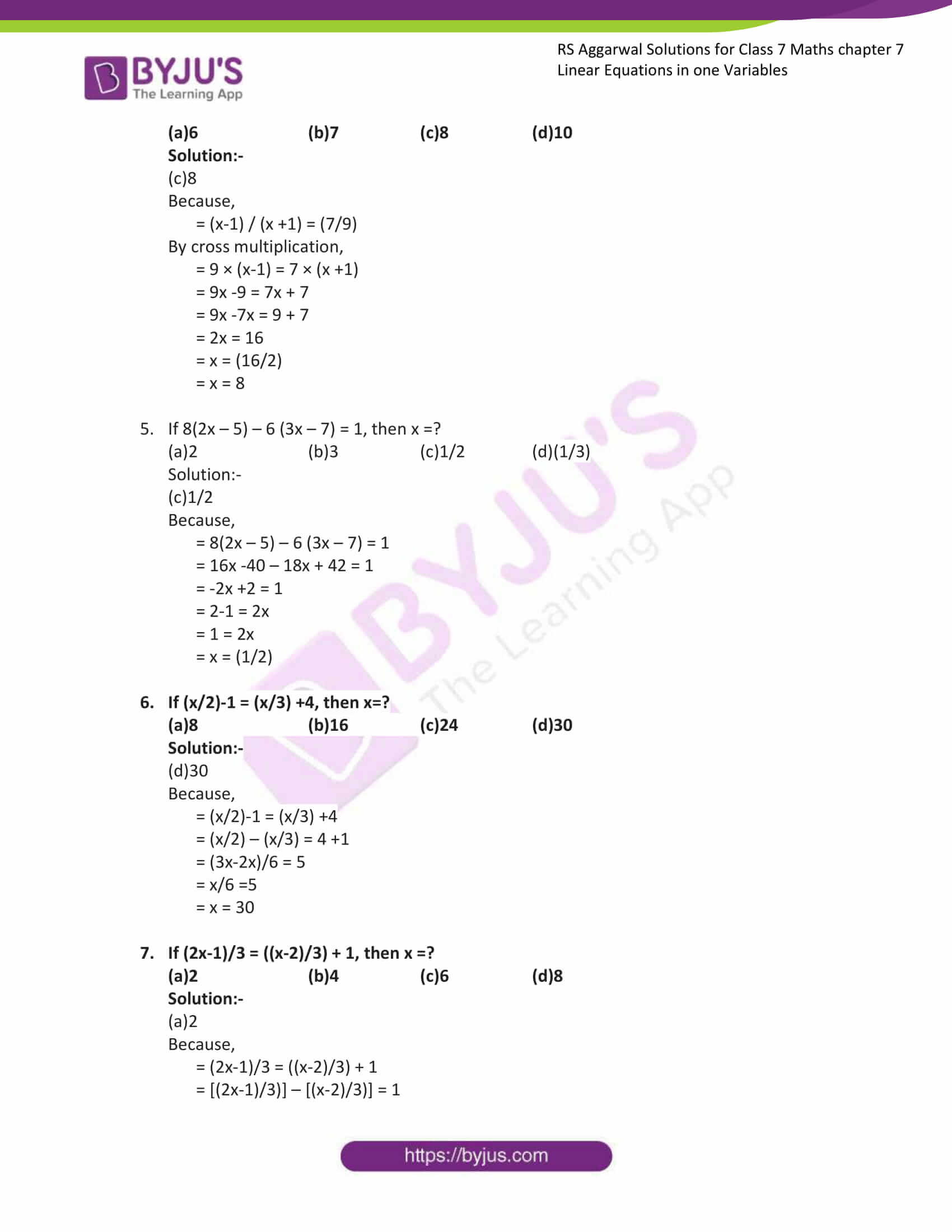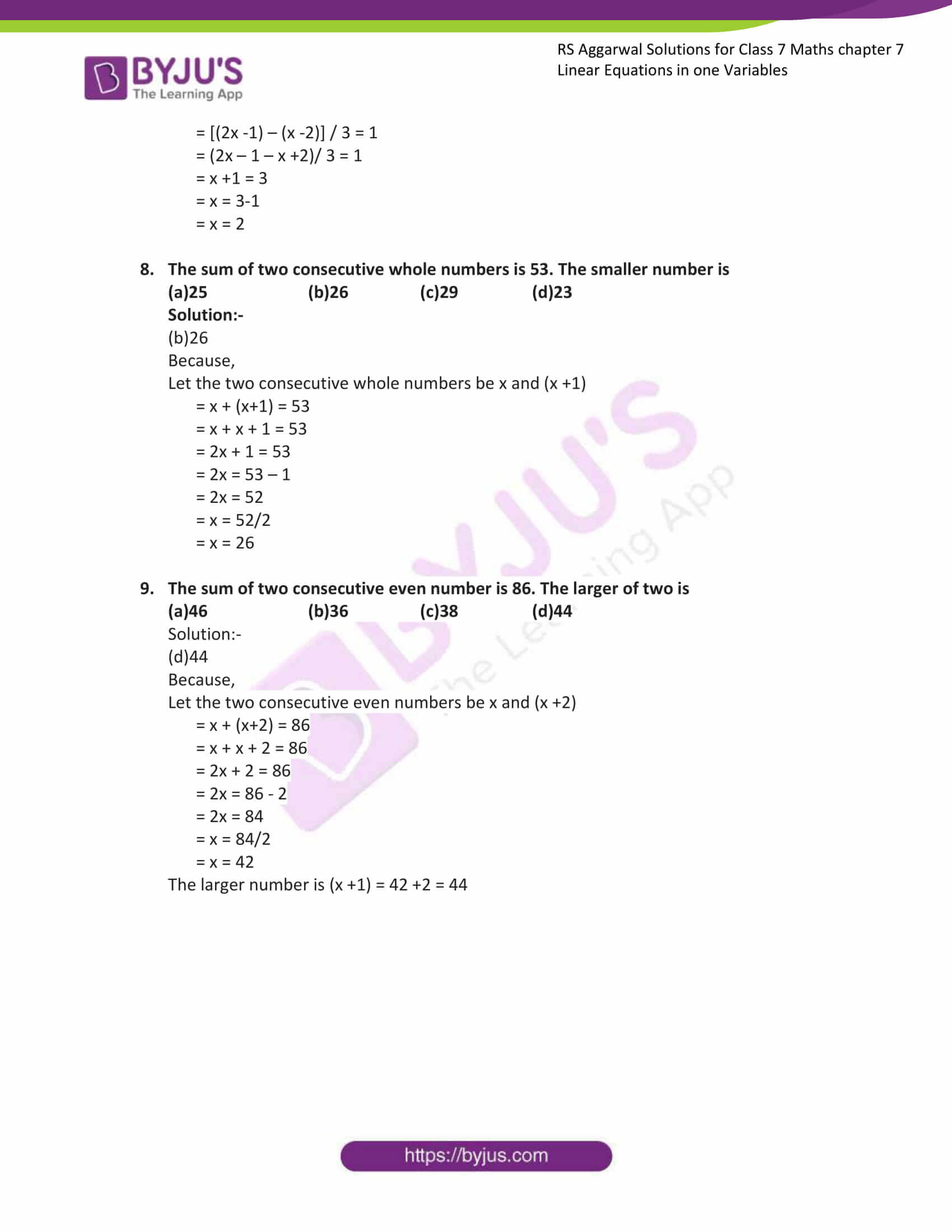Exercise 7A

Exercise 7B

Exercise 7C

## Exercise 7A

Solve the following equations. Check your result in each case.

1. 3x – 5 = 0Solution:-The value of the variable which makes the equation a true statement is called the root of the equation.Now, find the value of x by considering the given equation,

= 3x – 5 = 0

Transposing – 5 to RHS it becomes 5

= 3x = 5

Multiplying both side by (1/3)

= 3x × (1/3) = 5 × (1/3)

= x = (5/3)

By substituting (5/3) in the place of x in given equation, we get

= 3 × (5/3) – 5 = 0

= 5 – 5 = 0

= 0= 0

∴ LHS = RHS

Hence, the result is verified.

2. 8x – 3 = 9 – 2xSolution:-Now, find the value of x by considering the given equation,=8x – 3 = 9 – 2x

Transposing – 3 to RHS and it becomes 3 and -2x to LHS it becomes 2x

= 8x + 2x = 9 + 3

= 10x = 11

Multiplying both side by (1/10)

= 10x × (1/10) = 12 × (1/10)

= x = (12/10)

= x = (6/5)

By substituting (6/5) in the place of x in given equation, we get

LHS,

= 8 × (6/5) – 3

= (48/5) – 3

= (48-15/5)

= (33/5)

RHS,

= 9 – 2 × (6/5)

= 9 – (12/5)

= (45 – 12)/5

= (33/5)

By comparing LHS and RHS

= (33/5) = (33/5)

∴ LHS = RHS

Hence, the result is verified.

3. 7 – 5x = 5 – 7xSolution:-Now, find the value of x by considering the given equation,=7 – 5x = 5 – 7x

Transposing 7 to RHS and it becomes -7 and -7x to LHS it becomes 7x

= 7x – 5x = 5 – 7

= 2x = -2

Multiplying both side by (1/3)

= 2x × (1/2) = -2 × (1/2)

= x = (-2/2)

= x = -1

By substituting -1 in the place of x in given equation, we get

LHS,

= 7 – 5 × (-1)

= 7 – (-5)

= 7 + 5

= 12

RHS,

= 5 – 7 × (-1)

= 5 – (-7)

= 5 + 7

= 12

By comparing LHS and RHS

= 12 = 12

∴ LHS = RHS

Hence, the result is verified.

4. 3 + 2x = 1 – xSolution:-Now, find the value of x by considering the given equation,=3 + 2x = 1 – x

Transposing 3 to RHS and it becomes -3 and -x to LHS it becomes x

= 2x + x = 1 – 3

= 3x = -2

Multiplying both side by (1/3)

= 3x × (1/3) = -2 × (1/3)

= x = (-2/3)

By substituting -2/3 in the place of x in given equation, we get

LHS,

= 3 + 2 × (-2/3)

= 3 + (-4/3)

= 3 – (4/3)

= (9 – 4) / 3

= 5/3

RHS,

= 1 – (-2/3)

= 1 + (2/3)

= (3 + 2)/ 3

= 5/3

By comparing LHS and RHS

= 5/3 = 5/3

∴ LHS = RHS

Hence, the result is verified.

5. 2 (x – 2) + 3 (4x – 1) = 0Solution:-The above question can be written as,= 2x – 4 + 12x – 3 = 0

Now, find the value of x by considering the above equation,

= 2x + 12x – 4 – 3 = 0

= 14x – 7 = 0

Transposing -7 to RHS and it becomes 7

= 14x = 7

Multiplying both side by (1/14)

= 14x × (1/14) = 7 × (1/14)

= x = (7/14)

= x = 1/2

By substituting 1/2 in the place of x in given equation, we get

LHS,

= (2 × (1/2)) – 4 + (12 × (1/2)) – 3

= 1– 4 + 6 – 3

= 7 – 7

= 0

By comparing LHS and RHS

= 0 = 0

∴ LHS = RHS

Hence, the result is verified.

6. 5 (2x – 3) – 3 (3x – 7) = 5Solution:-The above question can be written as,= 10x – 15 – 9x + 21 = 5

Now, find the value of x by considering the given equation,

= 10x – 15 – 9x + 21 = 5

= x + 6 = 5

Transposing 6 to RHS and it becomes -6

= x = 5 – 6

= x = -1

By substituting -1 in the place of x in given equation, we get

LHS,

= 10 (-1) -15 -9(-1) + 21

= -10 – 15 + 9 + 21

= -25 + 30

= 5

By comparing LHS and RHS

= 5 = 5

∴ LHS = RHS

Hence, the result is verified.

7. 2x – (1/3) = (1/5) – xSolution:-Now, find the value of x by considering the given equation,=2x – (1/3) = (1/5) – x

Transposing – (1/3) to RHS and it becomes (1/3) and – x to LHS it becomes x

= 2x + x = (1/5) + (1/3)

= 3x = (3 + 5) / 15

= 3x = 8/15

Multiplying both side by (1/3)

= 3x × (1/3) = (8/15) × (1/3)

= x = (8/45)

By substituting (8/45) in the place of x in given equation, we get

LHS,

= 2 × (8/45) – (1/3)

= (16/45) – (1/3)

= (16 – 15) / 45

= (1/45)

RHS

= (1/5) – (8/45)

= (9-8) / 45

= (1/45)

By comparing LHS and RHS

= (1/45) = (1/45)

∴ LHS = RHS

Hence, the result is verified.

8. (1/2)x- 3 = 5 + (1/3)xSolution:-Now, find the value of x by considering the given equation,= (1/2)x- 3 = 5 + (1/3)x

Transposing -3 to RHS and it becomes 3 and (1/3)x to LHS it becomes (-1/3)x

= (1/2)x – (1/3)x = 5 + 3

= ((3-2)/6)x = 8

= (1/6)x = 8

Multiplying both side by 6

= 6 × (1/6)x = 8 × 6

= x = 48

By substituting 48 in the place of x in given equation, we get

LHS,

= (1/2) × (48) – 3

= (48/2) – 3

= 24 – 3

= 21

RHS,

= 5 + (1/3) × (48)

= 5 + (48/3)

= 5 + 16

= 21

By comparing LHS and RHS

= 21 = 21

∴ LHS = RHS

Hence, the result is verified.

9. (x/2) + (x/4) = (1/8)Solution:-Now, find the value of x by considering the given equation,= (x/2) + (x/4) = (1/8)

= ((2 + 1) / 4) = (1/8)

= (3/4)x = (1/8)

Multiplying both side by (4/3)

= (3/4)x × (4/3) = (1/8) × (4/3)

= x = (1/6)

By substituting (1/6) in the place of x in given equation, we get

LHS,

= (3/4)x

= (3/4) × (1/6)

= (1/8)

RHS,

= (1/8)

By comparing LHS and RHS

= (1/8) = (1/8)

∴ LHS = RHS

Hence, the result is verified.

10. 3x + 2(x + 2) = 20 – (2x – 5)Solution:-The above question can be written as,= 3x + 2x + 4 = 20 – 2x + 5

Now, find the value of x by considering the above equation,

= 5x + 4 = 20 – 2x + 5

Transposing 4 to RHS and it becomes -4 and -2x to LHS it becomes 2x

= 5x + 2x = 20 – 4 + 5

= 7x = 25 – 4

= 7x = 21

Multiplying both side by (1/7)

= 7x × (1/7) = 21 × (1/7)

= x = 21/7

= x = 3

By substituting 3 in the place of x in given equation, we get

LHS,

= 5x + 4

= 5 × (3) + 4

= 15 + 4

= 19

RHS,

= 20 – 2x + 5

= 20 – 2 × (3) + 5

= 20 – 6 + 5

= 25 – 6

= 19

By comparing LHS and RHS

= 19 = 19

∴ LHS = RHS

Hence, the result is verified.

11. 13( y – 4) – 3(y-9) – 5(y + 4) = 0Solution:-The above question can be written as,= 13y – 52 – 3y + 27 – 5y – 20 = 0

Now, find the value of y by considering the above equation,

= 13y – 3y – 5y – 52 + 27 – 20 = 0

= 13y – 8y – 72 + 27 = 0

= 5y – 45 = 0

Transposing -45 to RHS and it becomes 45

= 5y = 45

Multiplying both side by (1/5)

= 5y × (1/5) = 45 × (1/5)

= y = 9

By substituting 9 in the place of y in given equation, we get

LHS,

= 5y – 45

= 5 × (9) – 45

= 45 – 45

= 0

RHS,

= 0

By comparing LHS and RHS

= 0 = 0

∴ LHS = RHS

Hence, the result is verified.

12. (2m + 5) / 3 = (3m – 10)Solution:-The above question can be written as,= 3 × [(2m + 5)/ 3] = 3 × (3m -10) … [Multiplying both side by 3]

= (2m + 5) = 9m – 30

Transposing 2m to RHS and it becomes -2m and -30 to LHS it becomes 30

= 30 + 5 = 9m -2m

= 35 = 7m

Multiplying both side by (1/7),

= 7m × (1/7) = 35 × (1/7)

= m = (35/7)

= m = 5

By substituting 5 in the place of m in given equation, we get

LHS,

= 2m + 5

= 2 × (5) + 5

= 10 + 5

= 15

RHS,

= 9m – 30

= 9 × (5) – 30

= 45 – 30

= 15

By comparing LHS and RHS

= 15 = 15

∴ LHS = RHS

Hence, the result is verified.

13. 6(3x + 2) – 5(6x – 1) = 3(x – 8) – 5(7x – 6) + 9xSolution:-The above question can be written as,= 18x + 12 – 30x + 5 = 3x – 24 – 35x + 30 + 9x

Now, find the value of x by considering the above equation,

Transposing 12, 5 to RHS and it becomes -12, -5 and -35x, 9x, 3x to LHS it becomes 35x, -9x, -3x

= 18x – 30x + 35x – 9x – 3x = -24 + 30 – 12 – 5

= 53x – 42x = 30 – 41

= 11x = -11

Multiplying both side by (1/11),

= 11x × (1/11) = -11 × (1/11)

= x = (-11/11)

= x = -1

By substituting 5 in the place of x in given equation, we get

LHS,

= 18x + 12 – 30x + 5

= 18 (-1) + 12 – 30 (-1) + 5

= -18 + 12 + 30 + 5

= -18 + 47

= 29

RHS,

= 3x – 24 – 35x + 30 + 9x

= 3 (-1) – 24 – 35 (-1) + 30 + 9 (-1)

= -3 – 24 + 35 + 30 – 9

= -36 + 65

= 29

By comparing LHS and RHS

= 15 = 15

∴ LHS = RHS

Hence, the result is verified.

14. t – (2t + 5) – 5(1 – 2t) = 2(3 + 4t) – 3(t -4)Solution:-The above question can be written as,= t – 2t – 5 – 5 + 10t = 6 + 8t – 3t + 12

Now, find the value of t by considering the above equation,

Transposing -5, -5 to RHS and it becomes 5, 5 and 8t, -3t to LHS it becomes -8t, 3t

= t – 2t + 10t – 8t + 3t = 6 + 12 + 5 + 5

= 14t – 10t = 28

= 4t = 28

Multiplying both side by (1/4),

= 4t × (1/4) = 28 × (1/4)

= t = 7

By substituting 7 in the place of t in given equation, we get

LHS,

= t – 2t – 5 – 5 + 10t

= 7 – 2× (7) – 5 – 5 + 10(7)

= 7 – 14 – 5 – 5 + 70

= 77 – 24

= 53

RHS,

= 6 + 8t – 3t + 12

= 6 + 8 × (7) – 3 × (7) + 12

= 6 + 56 – 21 + 12

= 74 – 21

= 53

By comparing LHS and RHS

= 15 = 15

∴ LHS = RHS

Hence, the result is verified.

15. (2/3)x = (3/8)x + (7/12)Solution:-Find the value of x by considering the above equation,Transposing (3/8)x to RHS and it becomes -(3/8)x.

= (2/3)x – (3/8)x = (7/12)

= [(16 – 9) / 24]x = (7/12)

= (7/24) x = (7/12)

= x = 2

By substituting 2 in the place of x in given equation, we get

LHS,

= (2/3)x

= (2/3) × (2)

= (4/3)

RHS,

= (3/8)x + (7/12)

= (3/8) × (2) + (7/12)

= (3/4) + (7/12)

= (9 + 7) / (12)

= (16/12)

= (4/3)

By comparing LHS and RHS

= (4/3) = (4/3)

∴ LHS = RHS

Hence, the result is verified.

16. [(3x – 1)/ 5] – (x/7) = 3Solution:-The above question can be written as,= [(7 × (3x -1)) – (5 × (x))/35] = 3

= ((21x – 7) – (5x))/35 = 3

= (21x – 7 – 5x)/35 = 3

= (16x – 7)/35 = 3 … [Multiplying both side by 35]

= [(16x – 7)/35] × 35 = 3 × 35

= 16x – 7 = 105

= 16x = 105 + 7

= 16x = 112 … [Multiplying both side by 1/16]

= 16x × (1/16) = 112 × (1/16)

= x = 7

By substituting 7 in the place of x in given equation, we get

LHS,

= (16x – 7)/35

= ((16 × 7) – 7)/35

= (112 – 7)/ 35

= (105/35)

= 3

RHS,

= 3

By comparing LHS and RHS

= (4/3) = (4/3)

∴ LHS = RHS

Hence, the result is verified.

## Exercise 7B

1. Twice a number when decreased by 7 gives 45. Find the number.Solution:-Let the required number be x.Then,

= 2x – 7 = 45

Transposing -7 to RHS and it becomes 7.

= 2x = 45 + 7

= 2x = 52

Multiplying both side by (1/2)

= 2x × (1/2) = 52 × (1/2)

= x = 26

∴ the required number is 26

2. Thrice a number when increased by 5 gives 44. Find the number.Solution:-Let the required number be x.Then,

= 3x + 5 = 44

Transposing 5 to RHS and it becomes -5.

= 3x = 44 – 5

= 3x = 39

Multiplying both side by (1/3)

= 3x × (1/3) = 39 × (1/3)

= x = 13

∴ the required number is 13

3. Four added to twice a number yields (26/5). Find the fraction.Solution:-Let the required number be x.Then,

= 2x + 4 = (26/5)

Transposing 4 to RHS and it becomes -4.

= 2x = (26/5) – 4

= 2x = (26 – 20)/5

= 2x = 6/5

Multiplying both side by (1/2)

= 2x × (1/2) = (6/5) × (1/2)

= x = 3/5

∴ the required number is 3/5

4. A number when added to its half gives 72. Find the number.Solution:-Let the required number be x.Then,

= x + (x/2) = 72

= (2x + x)/ 2 = 72

= 3x/2 = 72

Multiplying both side by (2)

= (3x/2) × (2) = 72 × 2

= 3x = 144

Again, multiplying both side by (1/3)

= 3x × (1/3) = 144 × (1/3)

= x = 48

∴ the required number is 48

5. A number added to its two-thirds is equal to 55. Find the number.Solution:-Let the required number be x.Then,

= x + (2x/3) = 55

= (3x + 2x)/3 = 55

= 5x/3 = 55

Multiplying both side by (3/5)

= (5x/3) × (3/5) = 55 × (3/5)

= x= 11 × 3

= x = 33

∴ the required number is 33

6. A number when multiplied by 4, exceeds itself by 45. Find the number.Solution:-Let the required number be x.Then,

= 4x – x = 45

= 3x = 45

Multiplying both side by (1/3)

= 3x × (1/3) = 45 × (1/3)

= x = 15

∴ the required number is 15

7. A number is as much greater than 21 as it is less than 71. Find the number.Solution:-Let the required number be x.Then,

= (x – 21) = (71 –x)

Transposing -21 to RHS and it becomes 21 and –x to LHS it becomes x.

= x + x = 71 + 21

= 2x = 92

Multiplying both side by (1/2)

= 2x × (1/2) = 92 × (1/2)

= x = 46

∴ the required number is 46

8. (2/3) of a number is less than the original number by 20. Find the number.Solution:-Let the required number be x.Then,

= (2x/3) = x – 20

Transposing x to LHS and it becomes –x.

= (2x/3) – x = -20

= (2x – 3x)/3 = -20

= -x/3 = -20

Multiplying both side by – 3

= (-x/3) × -3 = -20 × -3

= x = 60

∴ the required number is 60

9. A number is (2/5) times another number. If their sum is 70. Find the numbers.Solution:-Let the required number be x.Then,

= x + (2x/5) = 70

= (5x + 2x)/5 = 70

= (7x/5) = 70

Multiplying both side by (5/7)

= (7x/5) × (5/7) = 70 × (5/7)

= x = 50

The other number is (2x/5)

= (2 × 50)/5

= 100/5

= 20

∴the required numbers are 50 and 20

10. Two- thirds of a number is greater than one-third of the number by 3. Find the number.Solution:-Let the required number be x.Then,

= (2x/3) = (1x/3) + 3

Transposing (1x/3) to LHS and it becomes – (1x/3)

= (2x/3) – (1x/3) = 3

= (2x – 1x)/ 3 = 3

= (1x/3) = 3

Multiplying both side by (3)

= (1x/3) × 3 = 3 × 3

= x = 9

∴the required numbers are 9

11. The fifth part of a number when increased by 5 equals its fourth part decreased by 5. Find the number.Solution:-Let the required number be x.Then,

= (x/5) + 5 = (x/4) – 5

Transposing (x/4) to LHS and it becomes – (x/4) and 5 to RHS it becomes -5

= (x/5) – (x/4) = -5 – 5

= (4x – 5x)/20 = -10

= -x/20 = -10

Multiplying both side by (-20)

= (-x/20) × (-20) = (-10) × (-20)

= x = 200

∴the required numbers are 200

12. Find two consecutive natural numbers whose sum is 63.Solution:-Let the required two consecutive natural numbers be x and (x + 1)Then,

= x + (x+1) = 63

= x + x + 1 = 63

= 2x + 1 = 63

Transposing 1 to LHS and it becomes – 1

= 2x = 63 -1

= 2x = 62

Multiplying both side by (1/2)

= 2x × (1/2) = 62 × (1/2)

= x = 31

The other number is (x + 1) = 31 + 1 = 32

∴the required two consecutive natural numbers are 31 and 32

13. Find two consecutive positive odd integers whose sum is 76Solution:-Let the required two consecutive positive odd integers be x and (x + 2)Then,

= x + (x+2) = 76

= x + x + 2 = 76

= 2x + 2 = 76

Transposing 2 to LHS and it becomes – 2

= 2x = 76 -2

= 2x = 74

Multiplying both side by (1/2)

= 2x × (1/2) = 74 × (1/2)

= x = 37

The other number is (x + 2) = 37 + 2 = 39

∴the required two consecutive natural numbers are 37 and 39

14. Find three consecutive positive even integers whose sum is 90Solution:-Let the required three consecutive positive even integers be x, (x + 2) and (x + 4)Then,

= x + (x+2) + (x+4) = 90

= x + x + 2 + x + 4 = 90

= 3x + 6 = 90

Transposing 6 to LHS and it becomes – 6

= 3x = 90 -6

= 3x = 84

Multiplying both side by (1/3)

= 3x × (1/3) = 90 × (1/3)

= x = 30

The other numbers are (x + 2) = 30 + 2 = 32 and (x + 4) = 30 + 4 = 34

∴the required three consecutive positive even integers are 30, 32 and 34

15. Divide 184 into two parts such that one-third of one part may exceed one- seventh of the other part by 8.Solution:-Let the two parts be x and (184 – x)Then,

= (1/3)x – (1/7) (184 – x) = 8

= (1/3)x – (184/7) + (x/7) = 8

Transposing – (184/7) to LHS and it becomes (184/7)

= (1/3) x + (x/7) = 8 + (184/7)

= [(7 + 3)/21]x = (56 +184)/7

= (10/21) x = 240/7

Multiplying both side by (21/10)

= (10/21)x × (21/10) = (240/7) × (21/10)

= x = (240 × 21)/ (7 × 10)

= x = (24 × 3)/ (1 × 1)

= x = 72

16. A sum of ₹ 500 is in the form of denominations of ₹ 5 and ₹ 10.if the total number of notes is 90. Find the number of notes of each type.Solution:-The total number of notes is 90.Let the number of ₹ 5 note be x

And the number of ₹ 10 note be (90 –x)

Then,

= 5x + 10(90 – x) = 500

= 5x + 900 -10x = 500

= – 5x + 900 = 500

Transposing – 5x to RHS and it becomes 5x and 500 to LHS it becomes -500

= 900 – 500 = 5x

= 400 = 5x

Multiplying both side by (1/5)

= 400 × (1/5) = 5x × (1/5)

= 80 = x

∴the number of ₹ 10 note be (90 –x) = (90 – 80) = 10 notes

17. Sumitra has ₹ 34 in 50-paise and 25-paise coins. If the number of 25-paise coins is twice the number of 50-paise coins, how many coins of each kind does she have?Solution:-From the question,Sumitra has ₹34 = 3400 paise

Let 50 paise coins be x

And 25 paise coins be 2x

Then,

= 50x + 25 (2x) = 3400

= 50x + 50x = 3400

= 100x = 3400

Multiplying both side by (1/100).

= 100x × (1/100) = (3400) × (1/100)

= x = 34

∴The number of 50 paise coins is 34

The number of 25 paise coins is 2x = 2 × 34 = 68

## Exercise 7c

Mark against the correct answer in each of the following:

1. If 5x – (3/4) = 2x – (2/3), then x =?(a)(1/12) (b)(1/4) (C) 36 (d) (1/36)Solution:-(d) (1/36)

Because,

Transposing – (3/4) to RHS and it becomes (3/4) and 2x to LHS it becomes -2x

= 5x – 2x = – (2/3) + (3/4)

= 3x = (-8+9)/12

= 3x = 1/12

Multiplying both side by (1/3).

= 3x × (1/3) = (1/12) × (1/3)

= x = 1/36

2. If 2z + (8/3) = (1/4) z + 5, then z =?(a)3 (b)3 (c)(2/5) (d)(4/3)Solution:-(d)(4/3)

Because,

Transposing (8/3) to RHS and it becomes – (8/3) and (1/4)z to LHS it becomes –(1/4)z

= 2z – (1/4)z = 5 – (8/3)

= [(8-1)/4]z = (15-8)/3

= (7/4)z = 7/3

Multiplying both side by (4/7).

= (7/4)z × (4/7) = ((7/3) × (4/7)

= x = 4/3

3. If (2n + 5) = 3(3n – 10), then z =?(a)5 (b)3 (c)(2/5) (d)(2/5)Solution:-(a)5

Because,

= 2n + 5 = 9n – 30

Transposing 2n to RHS and it becomes – 2n and -30 to LHS it becomes 30

= 30 + 5 = 9n -2n

= 35 = 7n

Multiplying both side by (1/7).

= 35 × (1/7) = 7n × (1/7)

= 5 = n

4. If (x-1) / (x +1) = (7/9), then x =?(a)6 (b)7 (c)8 (d)10Solution:-(c)8

Because,

= (x-1) / (x +1) = (7/9)

By cross multiplication,

= 9 × (x-1) = 7 × (x +1)

= 9x -9 = 7x + 7

= 9x -7x = 9 + 7

= 2x = 16

= x = (16/2)

= x = 8

5. If 8(2x – 5) – 6 (3x – 7) = 1, then x =?(a)2 (b)3 (c)1/2 (d)(1/3)Solution:-(c)1/2

Because,

= 8(2x – 5) – 6 (3x – 7) = 1

= 16x -40 – 18x + 42 = 1

= -2x +2 = 1

= 2-1 = 2x

= 1 = 2x

= x = (1/2)

6. If (x/2)-1 = (x/3) +4, then x=?(a)8 (b)16 (c)24 (d)30Solution:-(d)30

Because,

= (x/2)-1 = (x/3) +4

= (x/2) – (x/3) = 4 +1

= (3x-2x)/6 = 5

= x/6 =5

= x = 30

7. If (2x-1)/3 = ((x-2)/3) + 1, then x =?(a)2 (b)4 (c)6 (d)8Solution:-(a)2

Because,

= (2x-1)/3 = ((x-2)/3) + 1

= [(2x-1)/3)] – [(x-2)/3)] = 1

= [(2x -1) – (x -2)] / 3 = 1

= (2x – 1 – x +2)/ 3 = 1

= x +1 = 3

= x = 3-1

= x = 2

8. The sum of two consecutive whole numbers is 53. The smaller number is (a)25 (b)26 (c)29 (d)23Solution:-(b)26

Because,

Let the two consecutive whole numbers be x and (x +1)

= x + (x+1) = 53

= x + x + 1 = 53

= 2x + 1 = 53

= 2x = 53 – 1

= 2x = 52

= x = 52/2

= x = 26

9. The sum of two consecutive even number is 86. The larger of two is(a)46 (b)36 (c)38 (d)44Solution:-(d)44

Because,

Let the two consecutive even numbers be x and (x +2)

= x + (x+2) = 86

= x + x + 2 = 86

= 2x + 2 = 86

= 2x = 86 – 2

= 2x = 84

= x = 84/2

= x = 42

The larger number is (x +1) = 42 +2 = 44

## RS Aggarwal Solutions for Class 7 Maths Chapter 7 – Linear Equations in One Variables

Chapter 7 – Linear Equations in One Variables contains 3 exercises and the RS Aggarwal Solutions available on this page provide solutions to the questions present in the exercises. Now, let us have a look at some of the essential concepts discussed in this chapter.

• Linear Equations
• Problems Based on Linear Equations

### Chapter Brief of RS Aggarwal Solutions for Class 7 Maths Chapter 7 – Linear Equations in One Variables

RS Aggarwal Solutions for Class 7 Maths Chapter 7 – Linear Equations in One Variable. In this chapter, we study the meaning of an equation and also that of a linear equation. Meanwhile, also discuss the purpose of the solutions of a linear equation and two methods of obtaining it, One by trial-and-error and another by a systematic method involving addition, subtraction, multiplication or division of some non-zero number on both sides of the equation.At the end of the chapter, we study the formulation and solution of equations for some real-life problems.